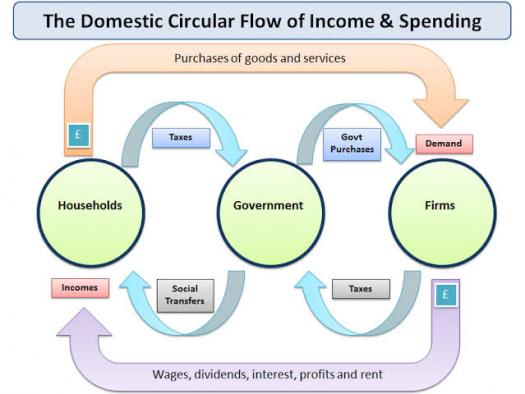# What Do You Know About Circular Flow Of Income?

10 Questions | Total Attempts: 103SettingsAs the name implies, the circular flow of income is a chart like structure which represents the income of a household, a city, or even a whole country. As its name implies, it looks like a circle. Let's test your knowledge on this subject by subjecting you to a few questions about it!

Related Topics
• 1.
The flows of money and services run in….
• A.

The same direction

• B.

Different directions

• C.

Parallel directions

• D.

Different directions but end up meeting

• 2.
How many models of a circular flow income chart exist?
• A.

Three

• B.

One

• C.

Four

• D.

Five

• 3.
What is the name of the last model?
• A.

Five sector model

• B.

Two sector model

• C.

One sector model

• D.

Three sector model

• 4.
What is a leakage in a circular flow of income chart?
• A.

When money gets into the chart

• B.

When money from the chart is invested

• C.

When money from the chart is spent recklessly

• D.

When money leaves the chart

• 5.
What constitute leakages?
• A.

Savings and Investments

• B.

Borrowing money

• C.

Lending money

• D.

Not doing anything at all with the money

• 6.
What is an injection in a circular flow of income chart?
• A.

When a household loses money

• B.

When money gets into the chart

• C.

When money is used for expenditure

• D.

When money from the chart is invested

• 7.
How many sectors exist in the circular flow of income chart?
• A.

One

• B.

Three

• C.

Five

• D.

Four

• 8.
What constitute injections?
• A.

Investments

• B.

Savings

• C.

Borrowing of money

• D.

Lending money

• 9.
All of these except one are part of the circular flow of income chart.
• A.

Household sector

• B.

Overseas sector

• C.

Government sector

• D.

International sector

• 10.
When is a state of equilibrium reached?
• A.

When the leakages surpass the injections

• B.

When injections surpass the leakages

• C.

When the leakages and injections are same

• D.

When there are no leakages nor injections